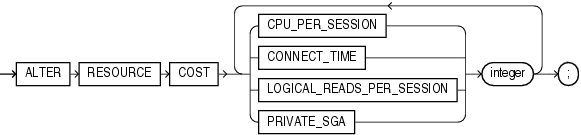## ALTER RESOURCE COST

Purpose

Use the `ALTER` `RESOURCE` `COST` statement to specify or change the formula by which Oracle Database calculates the total resource cost used in a session.

Although Oracle Database monitors the use of other resources, only the four resources shown in the syntax can contribute to the total resource cost for a session.

This statement lets you apply weights to the four resources. Oracle Database then applies the weights to the value of these resources that were specified for a profile to establish a formula for calculating total resource cost. You can limit this cost for a session with the `COMPOSITE_LIMIT` parameter of the `CREATE` `PROFILE` statement. If the resource cost of a session exceeds the limit, then Oracle Database aborts the session and returns an error. If you use the `ALTER` `RESOURCE` `COST` statement to change the weight assigned to each resource, then Oracle Database uses these new weights to calculate the total resource cost for all current and subsequent sessions.

CREATE PROFILE for information on all resources and on establishing resource limits

Prerequisites

You must have the `ALTER` `RESOURCE` `COST` system privilege.

Syntax

alter_resource_cost::=Description of the illustration alter_resource_cost.eps

Semantics

Oracle Database calculates the total resource cost by first multiplying the amount of each resource used in the session by the weight of the resource, and then summing the products for all four resources. For any session, this cost is limited by the value of the `COMPOSITE_LIMIT` parameter in the user's profile. Both the products and the total cost are expressed in units called service units.

CPU_PER_SESSION

Use this keyword to apply a weight to the `CPU_PER_SESSION` resource.

CONNECT_TIME

Use this keyword to apply a weight to the `CONNECT_TIME` resource.

Use this clause to apply a weight to the `LOGICAL_READS_PER_SESSION` resource. Logical reads include blocks read from both memory and disk.

PRIVATE_SGA

Use this clause to apply a weight to the `PRIVATE_SGA` resource. This limit applies only if you are using shared server architecture and allocating private space in the SGA for your session.

integer

Specify the weight of each resource. The weight that you assign to each resource determines how much the use of that resource contributes to the total resource cost. If you do not assign a weight to a resource, then the weight defaults to 0, and use of the resource subsequently does not contribute to the cost. The weights you assign apply to all subsequent sessions in the database.

Examples

Altering Resource Costs: Examples

The following statement assigns weights to the resources `CPU_PER_SESSION` and `CONNECT_TIME`:

```ALTER RESOURCE COST
CPU_PER_SESSION 100
CONNECT_TIME      1;
```

The weights establish this cost formula for a session:

```cost = (100 * CPU_PER_SESSION) + (1 * CONNECT_TIME)
```

In this example, the values of `CPU_PER_SESSION` and `CONNECT_TIME` are either values in the `DEFAULT` profile or in the profile of the user of the session.

Because the preceding statement assigns no weight to the resources `LOGICAL_READS_PER_SESSION` and `PRIVATE_SGA`, these resources do not appear in the formula.

If a user is assigned a profile with a `COMPOSITE_LIMIT` value of 500, then a session exceeds this limit whenever `cost` exceeds 500. For example, a session using 0.04 seconds of CPU time and 101 minutes of elapsed time exceeds the limit. A session using 0.0301 seconds of CPU time and 200 minutes of elapsed time also exceeds the limit.

You can subsequently change the weights with another `ALTER` `RESOURCE` statement:

```ALTER RESOURCE COST
CONNECT_TIME 0;
```

These new weights establish a new cost formula:

```cost = (100 * CPU_PER_SESSION) + (2 * LOGICAL_READ_PER_SECOND)
```

where the values of `CPU_PER_SESSION` and `LOGICAL_READS_PER_SECOND` are either the values in the `DEFAULT` profile or in the profile of the user of this session.

This `ALTER` `RESOURCE` `COST` statement changes the formula in these ways:

• The statement omits a weight for the `CPU_PER_SESSION` resource. That resource was already assigned a weight, so the resource remains in the formula with its original weight.

• The statement assigns a weight to the `LOGICAL_READS_PER_SESSION` resource, so this resource now appears in the formula.

• The statement assigns a weight of 0 to the `CONNECT_TIME` resource, so this resource no longer appears in the formula.

• The statement omits a weight for the `PRIVATE_SGA` resource. That resource was not already assigned a weight, so the resource still does not appear in the formula.